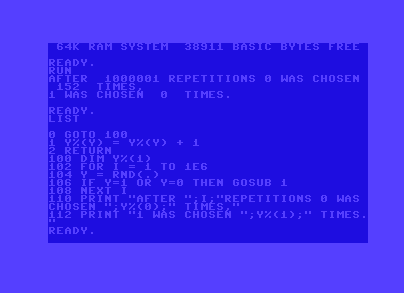# Talk:RND

## Random number between Zero and One

Is it really (0.0 to 1.0) or actually (0 to 0.999999999999)? If it's really (0.0 to 1.0) then there is an astronomically small POSSIBILITY that when you multiply, say, RND(0)*100 (if you want 0...99) you COULD get 100 eventually. It could lead to error in some cases. So which one is it? (let's say it is true (0.0-1.0) - then the best solution would be to make it calculate again if the result is 100 in that freak case, or put 99.999 instead of 100 - for example: instead of RND(0)*100 put RND(0)*99.999 - then it would be (0 (0.000-0.999) to 99 (99.000-99.999)) - equal chances for all numbers; but anyhow - the best is to definitely know if it's really (0.0 to 1.0) or actually (0 to 0.999999999999) - then it wouldn't be needed to put that freak-case-do-it-again program line at all)Moiree 01:11, 7 September 2013 (CEST)
If that emulator works exactly the same as the real C64 then it means that the claim in the article is wrong (RND gives numbers in range of 0 - 0.9999999...?, not 0.0 to 1.0). One could also make a one-line program like this: "0 if rnd(0)<>1 then 0" - so if program stops it means that 1 happened (also - does it matter what parameter is set for RND - 0/+-1? and isn't RND(.) the same as RND(0.0)?).
The german article says it better, the numbers are in the range of 0.0 (inclusive) and 1.0 (exclusive).
Yes, the function argument does matter, someone should add that to the article.
Yes, "." is the same as "0" or "0.0" for that matter, but is executed faster. BASIC is parsed at run time.
My program above is flawed though, as rnd(0) returns only random 256 bits, I changed the program accordingly and now neither zero nor one is chosen after 10^6 loops. Meh.
``` 0 goto 100
1 z(y) = z(y) + 1
2 return

100 dim z(1)
y = rnd(.)
for i = 1 to 1e6
y = rnd(y)
if y=1 or y=. then gosub 1
next i
print "after ";i;"repetitions 0 was chosen ";z(0);" times,"
print "1 was chosen ";z(1);" times."
```
Moiree 15:36, 8 September 2013 (CEST)